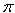SEARCH HOMEMath Central Quandaries & QueriesSubject: Yardage of dirt fill Name: Gene Who are you: Other I am removing dirt then replacing it with good dirt. I need to know how many yards of fill dirt it would take to fill a diameter 165 feet with fill of 13 ft would take how many cubic yards of dirt.Wow! That's a big hole.

You have a cylindrical hole that is 165 feet in diameter and 13 feet deep. Since you want the volume in cubic yards you should convert all the dimensions to yards. There are 3 feet in a yard so

165 ft = 165/3 yards = 55 yards
13 ft = 13/3 yards = 4.33 yards

The volume of a cylinder is

volume =r2 h

where r is the radius (half the diameter) and h is the height. Thus you have a volume of(55/2)24.33 = 10,287 cubic yards.

PennyMath Central is supported by the University of Regina and The Pacific Institute for the Mathematical Sciences.